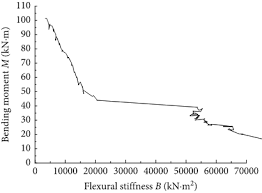## How to Calculate and Solve for Stiffness Performance Index | Material SelectionThe image above represents stiffness performance index.

To compute for stiffness performance index, two essential parameters are needed and these parameters are Shear Modulus (G) and Density (ρ).

The formula for calculating stiffness performance index:

P = √(G)/ρ

Where:

P = Stiffness Performance Index
G = Shear Modulus
ρ = Density

Let’s solve an example;
Find the stiffness performance index when the shear modulus is 36 and the density is 12.

This implies that;

G = Shear Modulus = 36
ρ = Density = 12

P = √(G)/ρ
P = √(36)/12
P = 6/12
P = 0.5

Therefore, the stiffness performance index is 0.5

Calculating the Shear Modulus when the Stiffness Performance Index and the Density is Given.

G = (P x ρ)2

Where:

G = Shear Modulus
P = Stiffness Performance Index
G = Shear Modulus= Stiffness Performance Index
ρ = Density

Let’s solve an example;
Find the shear modulus when the stiffness performance index is 25 and the density is 5.

This implies that;

P = Stiffness Performance Index = 25
ρ = Density = 5

G = (P x ρ)2
G = (25 x 5)2
G = 15,625

Therefore, the shear modulus is 15,625.